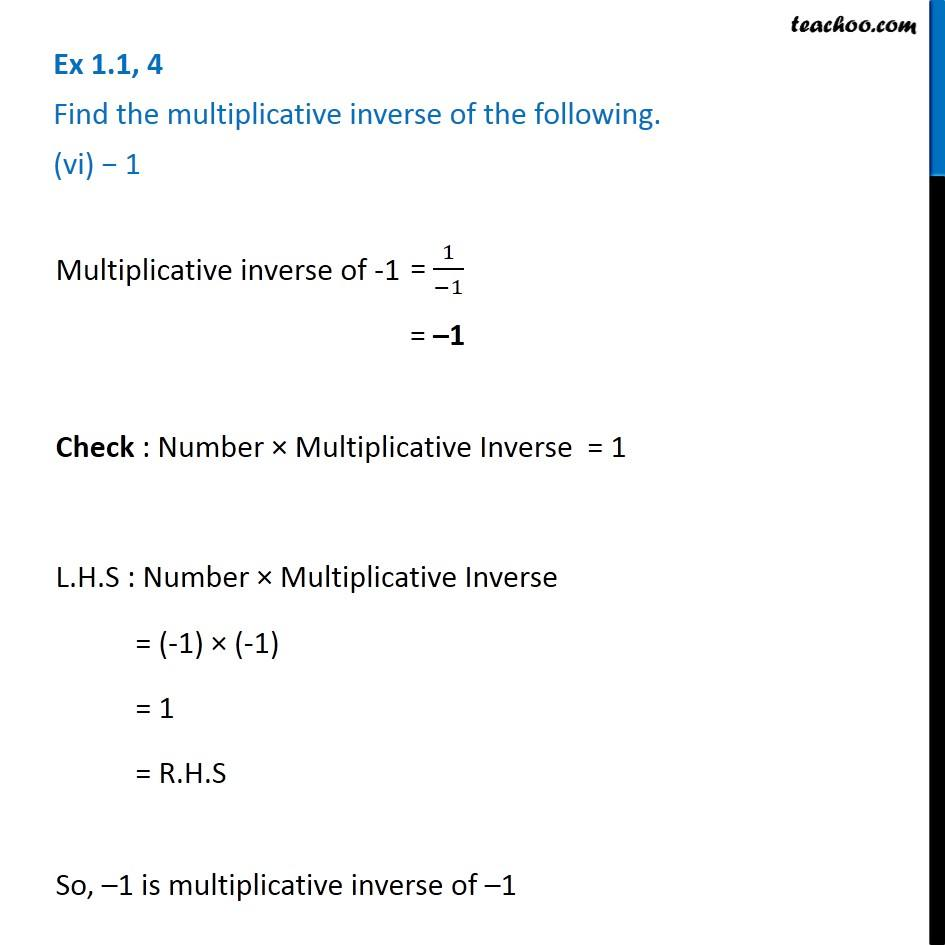Ex 1.1

Chapter 1 Class 8 Rational Numbers
Serial order wiseGet live Maths 1-on-1 Classs - Class 6 to 12

### Transcript

Ex 1.1, 4 Find the multiplicative inverse of the following. (vi) 1 Multiplicative inverse of -1 = 1 Check : Number Multiplicative Inverse = 1 L.H.S : Number Multiplicative Inverse = (-1) (-1) = 1 = R.H.S So, 1 is multiplicative inverse of 1